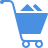Cart (0)

It measures the percentage of variation around the arithmetic mean of a series, and is calculated as follows:

Coefficient of Variation = Standard Deviation / Mean

It is commonly used as a measure of volatility in demand to assess the predictability of a demand pattern, that is, how well it can be forecasted. If COV >1.0, it can be said that variations in demand are high, and thus, statistical techniques should not be applied without further review.

Previous Entry